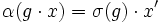# Equivalence of definitions of subgroup-conjugating automorphism

This article gives a proof/explanation of the equivalence of multiple definitions for the term subgroup-conjugating automorphism
View a complete list of pages giving proofs of equivalence of definitions

## The definitions that we have to prove as equivalent

### Subgroup-conjugating automorphism

An automorphism$\sigma$ of a group$G$ is termed a subgroup-conjugating automorphism if, for any subgroup$H$ of$G$,$H$ and$\sigma(H)$ are conjugate subgroups.

### Permutation-extensible automorphism

An automorphism$\sigma$ of a group$G$ is termed a permutation-extensible automorphism if, for any injective homomorphism$i: H \to \operatorname{Sym}(S)$, where$\operatorname{Sym}(S)$ is the symmetric group on a set, there is a permutation$\alpha$ of$S$ such that$i \circ \sigma = c_\alpha \circ i$, where$c_\alpha$ denotes conjugation by$\alpha$.

### Permutation-pushforwardable automorphism

An automorphism$\sigma$ of a group$G$ is termed a permutation-extensible automorphism if, for any homomorphism (not necessarily injective)$\rho: H \to \operatorname{Sym}(S)$, where$\operatorname{Sym}(S)$ is the symmetric group on a set, there is a permutation$\alpha$ of$S$ such that$\rho \circ \sigma = c_\alpha \circ \rho$, where$c_\alpha$ denotes conjugation by$\alpha$.

## Proof

### Permutation-extensible implies subgroup-conjugating

In this proof, we use the notation$c_g$ for conjugation by$g$, which is the map$x \mapsto gxg^{-1}$.

Given: A group$G$, a subgroup$H$, a permutation-extensible automorphism$\sigma$ of$G$.

To prove:$\sigma(H)$ is a conjugate subgroup to$H$.

Proof: The case that$H$ is the trivial subgroup is obvious, so we give the proof for$H$ nontrivial.

Let$S = G/H$ and$T = G$, with$G$ acting on$S$ and$T$ both by left multiplication. Let$U$ be the disjoint union of$S$ and$T$.$G$ acts faithfully on$U$, so we have an embedding:$G \to \operatorname{Sym}(U)$.

By the condition, there exists$\alpha \in \operatorname{Sym}(U)$ such that$\sigma$ extends to conjugation by$\alpha$ in$\operatorname{Sym}(U)$. Consider the element$H \in U$. Clearly, the isotropy subgroup of the element$\alpha H \in U$ is the subgroup$c_\alpha(H)$ in$G$, which equals$\sigma(H)$.

Now, observe that$\alpha H$ cannot be in$T$, because then its isotropy group would be trivial, and$\sigma(H)$ cannot be trivial if$H$ is nontrivial. Thus,$\alpha H \in S$, so there exists$g \in G$ such that$\alpha H = gH$. Thus, the isotropy subgroup of$\alpha(H)$ is the conjugate subgroup$c_g(H)$, and thus$\sigma(H) = c_g(H)$.

### Subgroup-conjugating implies permutation-pushforwardable

Given: A group$G$, an automorphism$\sigma$ of$G$ such that$\sigma$ sends every subgroup to a conjugate subgroup. A homomorphism$\rho:G \to \operatorname{Sym}(S)$ for some set$S$.

To prove: There exists a permutation$\alpha$ of$S$ such that$\rho \circ \sigma = c_\alpha \circ \rho$.

Proof: Let$\mathcal{O}$ be the orbit of some point in$S$ under the induced action of$G$. Let$x \in \mathcal{O}$, and let$H$ be the isotropy subgroup of$x$. Let$x'$ be any point in$\mathcal{O}$ whose isotropy subgroup is$\sigma(H)$. Such a point exists because$H$ and$\sigma(H)$ are conjugate subgroups. Now define:$\alpha(g \cdot x) = \sigma(g) \cdot x'$.

This is well-defined and gives a permutation of the orbit$\mathcal{O}$. If we define$\alpha$ in this way for each orbit, we get a permutation of$S$ and it satisfies the condition$\rho \circ \sigma = c_\alpha \circ \rho$.

### Puermutation-pushforwardable implies permutation-extensible

This implication is obvious.